mscroggs.co.uk
mscroggs.co.uksubscribe

# Blog

2021-05-22
In this post I'd like to talk about the catchily named Stirling numbers of the second kind, which I first encountered in this Maths Stack Exchange post. I'll start with some motivation for Stirling numbers of the second kind, show how they can be recursively calculated, and then show some interesting features of these numbers.
Suppose a fair die has $$f$$ distinct faces, and suppose you roll it $$n$$ times. What's the probability that you roll exactly $$k$$ distinct faces? Since the die is fair, each sequence of rolls is equally likely, so the probability is given by
$$\frac{\text{number of ways to roll the die $$n$$ times and observe $$k$$ distinct faces}}{\text{number of sequences of $$n$$ rolls}}.$$
The number of possible sequences of $$n$$ rolls is just $$f^{n}$$. And since, if we see exactly $$k$$ distinct faces, the $$k$$ distinct faces are equally likely to be any of the subsets of size $$k$$ out of $$f$$. So we have:
$$\begin{array}{lr} \text{ways to observe $$k$$ distinct faces in $$n$$ rolls}\hspace{-7cm}&\\[-7mm]&= (\text{ways to observe $$\{1, 2, \dots, k\}$$ in $$n$$ rolls}) \times \displaystyle\binom{f}{k}. \end{array}$$
Let's think about breaking down the $$n$$ rolls into $$k$$ subsets based on which rolls matched each face. For example, if $$k = 3$$ and the sequence of rolls was $$\{1, 2, 1, 2, 3, 1\}$$, then the three subsets would be $$\{1, 3, 6\}, \{2, 4\}$$, and $$\{5\}$$, where the first subset is $$\{1, 3, 6\}$$ because rolls 1, 3, and 6 were face 1. Since each of the $$k$$ faces was observed, each subset must be non-empty. Any permutation of these subsets still creates a valid sequence of observations, e.g. if we swapped the first two subsets then the subsets would correspond to the sequence of rolls $$\{2, 1, 2, 1, 3, 2\}$$. And since there are $$k!$$ to permute the subsets, we have that the probability is
$$(\text{ways to partition $$n$$ items into $$k$$ non-empty subsets}) \times \binom{f}{k} \times k! \times f^{-n}.$$
The number of ways to partition $$n$$ items into $$k$$ non-empty subsets is a Stirling number of the second kind, denoted $$\left\{n\atop k\right\}$$.
In the same way that the factorial function is technically defined recursively, $$x! = \begin{cases} 1 & \text{if } x = 0,\\ (x - 1)! \thinspace x & \text{if } x > 0, \end{cases}$$ we can define the Stirling number of the second kind recursively. If $$n = 0$$ and $$k = 0$$, then the probability of seeing $$k$$ distinct numbers in $$n$$ rolls is 1, so we set $$\left\{0\atop 0\right\} = 1$$. If $$n \geq 1$$ then we are guaranteed to see at least $$1$$ distinct face, so $$\left\{n\atop 0\right\} = 0$$. If $$n = 0$$ and $$k\geq1$$, then seeing $$k$$ faces is impossible, so $$\left\{0\atop k\right\} = 0$$. And if $$k > n$$ then seeing $$k$$ distinct faces in $$n$$ rolls is impossible, so $$\left\{n\atop k\right\} = 0$$. These give us the base cases for a recursion. The recursive formula for $$n, k \geq 1$$ is given by
$$\left\{n\atop k\right\} = \left\{n - 1\atop k-1\right\} + k \left\{n - 1\atop k\right\}.$$
To see why, consider splitting $$n$$ items into $$k$$ non-empty subsets, and suppose that $$n - 1$$ of the items have already been added to subsets. We will consider two cases. In one case, the $$n - 1$$ items have only been assigned to $$k - 1$$ subsets (making each of these $$k-1$$ subsets non-empty) and we are forced to use the $$n$$th item to make the $$k$$th subset non-empty. There are $$\left\{n-1\atop k-1\right\}$$ ways that $$n-1$$ items can be assigned to make $$k-1$$ non-empty subsets, hence the first term in the sum. In the second case, the $$n-1$$ items have already been assigned to all $$k$$ subsets (making each of the $$k$$ subsets non-empty), and we are free to choose which of the $$k$$ subsets to put the $$n$$th item in. There are $$\left\{n-1\atop k\right\}$$ ways that $$n-1$$ items can be assigned to make $$k$$ non-empty subsets, and $$k$$ choices for the $$n$$th item, hence the second term in the sum.
The plot shows a "Pascal's triangle" for the Stirling numbers of the second kind, which I call "Stirling's second triangle". The top square is $$\left\{0\atop 0\right\}$$, the next row contains $$\left\{1\atop 0\right\}, \left\{1\atop 1\right\}$$, and so on.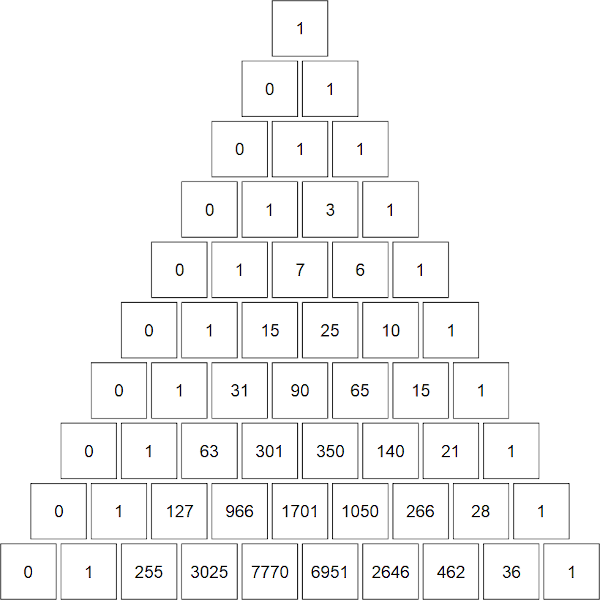The top ten rows of "Stirling's second triangle"
You can see how the generating rule differs from the one for $$\binom nk$$. Instead of $$\binom{n}{k} = \binom{n - 1}{k - 1} + \binom{n - 1}{k}$$ we have our recursive formula. Let's do the 5th row (corresponding to $$n = 4$$) as an example. We know from the base cases that $$\left\{4\atop 0\right\} = 0$$. Then $$\left\{4\atop 1\right\} = 0 + 1 \times 1 = 1$$, $$\left\{4\atop 2\right\} = 1 + 2 \times 3 = 7$$, $$\left\{4\atop 3\right\} = 3 + 3 \times 1 = 6$$, and $$\left\{4\atop 4\right\} = 1 + 4 \times 0 = 1$$.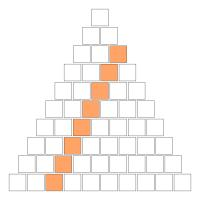The diagonal $$\left\{2\atop 2\right\}$$, $$\left\{3\atop 2\right\}$$, $$\left\{4\atop 2\right\}$$, $$\left\{5\atop 2\right\}$$, $$\left\{6\atop 2\right\}$$, ...
The diagonals in this triangle have some interesting features. Consider the diagonal $$\left\{2\atop 2\right\}$$, $$\left\{3\atop 2\right\}$$, $$\left\{4\atop 2\right\}$$, $$\left\{5\atop 2\right\}$$, $$\left\{6\atop 2\right\}$$, ... = 1, 3, 7, 15, 31, ... = $$2^1 - 1$$, $$2^2 - 1$$, $$2^3 - 1$$, $$2^4 - 1$$, $$2^5 - 1$$, ... The triangular numbers 0, 1, 3, 6, 10, 15, ... also make an appearance. I will leave it as an exercise for the reader to show that
$$\left\{n\atop 2\right\} = 2^{n-1} - 1,$$$$\text{ and } \left\{n\atop n-1\right\} = \binom{n}{2}.$$
Finally, an interesting feature occurs if you shade in the "Stirling's second triangle" according to the parity of the entry. Let the odd numbers be shaded grey and the white numbers be shaded white. At first it is difficult to discern a pattern, but it a fractal pattern related to the Sierpiński triangle emerges.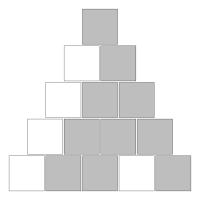The top five rows of "Stirling's second triangle" coloured by parity.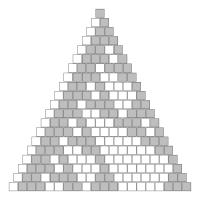The top twenty rows of "Stirling's second triangle" coloured by parity.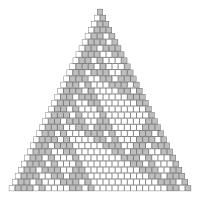The top thirty rows of "Stirling's second triangle" coloured by parity.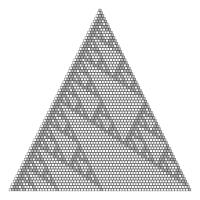The top sixty-six rows of "Stirling's second triangle" coloured by parity.

### Similar posts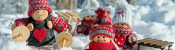Christmas (2020) is over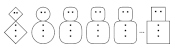Christmas card 2020Christmas (2020) is coming!Happy e+τ-5 Approximation Day!

Comments in green were written by me. Comments in blue were not written by me.

Allowed HTML tags: <br> <a> <small> <b> <i> <s> <sup> <sub> <u> <spoiler> <ul> <ol> <li>
To prove you are not a spam bot, please type "rotcaf" backwards in the box below (case sensitive):
2021-01-03
It's 2021, and the Advent calendar has disappeared, so it's time to reveal the answers and annouce the winners. But first, some good news: with your help, Santa received all his letters and Christmas was saved!
Now that the competition is over, the questions and all the answers can be found here. Before announcing the winners, I'm going to go through some of my favourite puzzles from the calendar, reveal the solution and a couple of other interesting bits and pieces.

### Highlights

My first highlight is this puzzle from 5 December, that I think doesn't seem obvious that there's even a unique answer until you spot some nice properties of dice.

### 5 December

Carol rolled a large handful of six-sided dice. The total of all the numbers Carol got was 521. After some calculating, Carol worked out that the probability that of her total being 521 was the same as the probability that her total being 200. How many dice did Carol roll?

My next highlight is the puzzle from 15 December. I had a lot of fun trying to write this one. At the end, I tell you that T represents 4: this puzzle still has a unique answer without this, but it was too hard to get started.

### 15 December

When talking to someone about this Advent calendar, you told them that the combination of XMAS and MATHS is GREAT. They were American, so asked you if the combination of XMAS and MATH is great; you said SURE. You asked them their name; they said SAM.
Each of the letters E, X, M, A, T, H, S, R, U, and G stands for a different digit 0 to 9. The following sums are correct:
Today's number is SAM. To help you get started, the letter T represents 4.

My next highlight is the puzzle from 16 December. I had a lot of fun writing this one too. At least a few people seem to have enjoyed solving it, as indicated by this comment.

### 16 December

Solve the crossnumber to find today's number. No number starts with 0.

My final highlight is the puzzle from 21 December. My method for solving this one was quite complicated. Let me know in the comments or on Twitter if you have a better way of solving it.

### 21 December

There are 3 ways to order the numbers 1 to 3 so that no number immediately follows the number one less that itself:
• 3, 2, 1
• 1, 3, 2
• 2, 1, 3
Today's number is the number of ways to order the numbers 1 to 6 so that no number immediately follows the number one less that itself.

### Hardest and easiest puzzles

Once you've entered 24 answers, the calendar checks these and tells you how many are correct. I logged the answers that were sent for checking and have looked at these to see which puzzles were the most and least commonly incorrect. The bar chart below shows the total number of incorrect attempts at each question.
You can see that the most difficult puzzles were those on 6, 14, 19, 21, and 24 December; and the easiest puzzles were on 3, 9, 10, 16, and 17 December.

### The solutions

The solutions to all the individual puzzles can be found here. If you want to read some alternative solutions to the puzzles, you can find Alira's solutions here, including a very nice explanation of how the final clues fit together to find the directions to Santa's house.
Using the daily clues, it was possible to work out that Santa's house could be found by following the directions 3,7,1,1,4,3,3,4,9,3,3,9 on this map.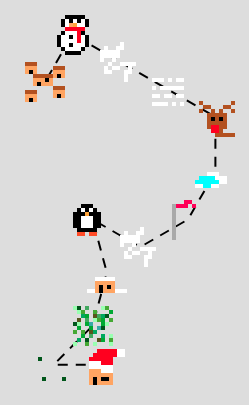Directions to Santa's house.
Due to the way the Advent compass worked, there are actually a few different ways to get to Santa's house. For example, 7,5,7,1,1,4,3,3,4,9,3,3 also gets you there: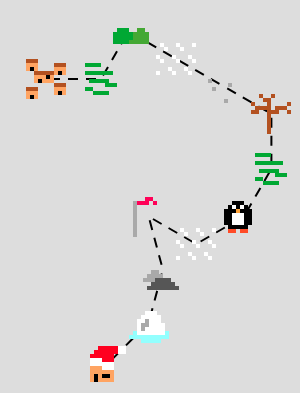Alternative directions to Santa's house.
I'll leave you to work out how many different ways there are to get there...
Here's the full collection of tiles that you could find by walking around the map: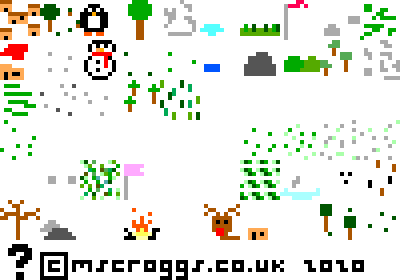The map tiles.

### The winners

And finally (and maybe most importantly), on to the winners: 223 people found Santa's house entered the competition. This is quite a lot more than in previous years:
From the correct answers, the following 10 winners were selected:
 1 Mars He 2 Ben Baker 3 Amelia Taylor 4 Alex Ayres 5 Hannah Charman 6 Diane Keimel 7 Alex Burlton 8 Rob March 9 Mahmood Hikmet 10 Guillermo Heras Prieto
Congratulations! Your prizes will be on their way shortly.
The prizes this year include 2020 Advent calendar T-shirts. If you didn't win one, but would like one of these, I've made them available to buy on Teespring alongside the T-shirts from previous years.
Additionally, well done to 4nder, Ashley Frazer Jarvis, Aaron Stiff, Alan Buck, Alejandro Villarreal, Alex Bolton, Alex Davis, Alex Klapheke, Alexander Rakitin, Anders Kaseorg, Andrew, Andrew P Turner, Andy Ennaco, Annabel, Anthony Della Pella, Artie Smith, Athena, Austin, Austin Shapiro, Beau Ferguson, Becky Russell, Ben Buchwald, Ben Jones, Ben Reiniger, Benjamin Tozer, Bennet, Beth Jensen, Blake, Brennan Dolson, Brian Carnes, Brian Wellington, Burak Kadron, Caleb Likely, Callum Hobbis, Carl Westerlund, Carmen Günther, Cath Simpson, Cathy Hooper, Chris Hellings, Christy Hales, Colin, Colin Brockley, Connie, Corbin Groothuis, Cory Peters, Cosmo Viola, Dan Colestock, Dan DiMillo, Dan McIntyre, Dan Whitman, Dana Sussman, Daniel De la Paz, David, David Ault, David Berardo, David Fox, David Kendel, David Mitchell, David Parmenter, David and Ivy Walbert, Dean Thomas, Deborah Tayler, Don Anderson, Donagh Collins, Elizabeth Blackwell, Emilie Heidenreich, Emily Troyer, Eric Kolbusz, Eric Skoglund, Erik Eklund, Eva, Evan Louis Robinson, Fionn Woodcock, Frances Haigh, Frank Kasell, Franklin Ta, Fred Verheul, Georges, Gabriella Pinter, Gert-Jan de Vries, Graham Greve, Gregory Loges, Gwendal Collet, Harry Allen, Heerpal Sahota, Helen, Helen Matthews, Herschel Pecker, Håkon Balteskard, Ivan Arribas, Jacob Young, James Demers, James Laver, Jan, Jason Demers, Jason Kass, Jean-Sébastien Turcotte, Jeff Rubin, Jefff Michael, Jen Huang, Jessica Marsh, Jim Rogers, Joe Gage, Johan Asplund, John Alasdair Warwicker, Jon Foster, Jon Lipscombe, Jon Palin, Jonathan Chaffer, Jonathan Forsythe, Jonathan Winfield, Jordan Susskind, Jorge del Castillo, Kai Lam, Karen Climis, Keith Pound, Ken Cheung, Killeen, Kristen Koenigs, Kristin Gramza, Kurt Engleman, Laura Midgley, Lauren Woolsey, Lewis Dyer, Linus Banghart-Linn, Liz Madisetti, Louis de Mendonca, Magnus Eklund, Mark Stambaugh, Martijn O., Martin Harris, Martin Holtham, Martine Nome, Matt Askins, Matt Hutton, Matthew Reynier, Matthew Riggle, Matthew Schulz, Matthew Scroggs, Matthias Planitzer, Maxime Rivet, Maximilian Pfister, Michael DeLyser, Michael Prescott, Mihai Zsisku, Mike Hands, Moritz Stocker, Nadine Chaurand, Nathan Chun, Nick C, Nick Keith, Nick Mohr, Norvin Richards, Olov Wilander, Pamela Docherty, Pau Batlle, Paul Livesey, Pranshu Gaba, Ray Arndorfer, Rea, Reuben Cheung, Riccardo Lani, Richard Pemberton, Rileigh Luczak, Road White, Roni, Rosie Paterson, Russ Collins, Ruth Franklin, Ryan Coomer, Ryan Seldon, Ryan Wise, Sam Dreilinger, Sam Hartburn, Sammie Buzzard, Sara Van Hoy, Sarah Brook, Saul Freedman, Scott, Sean, Sean Carmody, Sean Henderson, Sean McDonald, Sebastian, Selina Glauert, Seth Cohen, Shivanshi Adlakha, Simon Schneider, Sophie Maclean, Spencer B., Stephen Cappella, Stephen Dainty, Stephen Jasina, Steve Blay, Steve Paget, Steven Richardson, Susana Early, Tarim, Tim Holman-Wilkens, Tim Lewis, Todd Geldon, Tom Fryers, Tony Mann, Tristan Shephard, Valentin Valciu, Vinesh, Yasha, Yuliya Nesterova, Yurie Ito, and Zachary Polansky who all also found Santa's house but were too unlucky to win prizes this time.
See you all next December, when the Advent calendar will return.

### Similar postsChristmas (2020) is coming!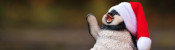Christmas (2019) is overChristmas (2019) is coming!Christmas (2018) is over

Comments in green were written by me. Comments in blue were not written by me.
Dec 15th was my favorite. I kept making a logical error and had to restart, so it took me way to long, but I really enjoyed it.

The 16th was just cheeky, after I spent way to much time on the 15th it was nice to have something like that!

It's been 16+ years since I did any probability or combinations and permutations, so it was nice to brush off that part of my brain, not that I did any of them well, but it should serve me well when my kids start doing them and ask me for help.
Dave
You can solve the Dec 21 puzzle using the principle of inclusion/exclusion:

-There are 6! total ways of arranging 6 numbers.
-Now we have to exclude the ones that don't fit. How many ways have 2 following 1? You can think of 12 as a pair, so you're arranging 12/3/4/5/6 in any order, so there are 5! ways to do this. And there are (5 choose 1)=5 total pairs that might exist, so there are 5*5! ways that have either 12, 23, 34, 45, or 56.
-Of course, we've double counted some that have more than one pair. (This is where inclusion/exclusion comes in, we have to include them back in). So how many have, say, 12 and 45? Well now we're arranging 12/3/45/6, so there are 4! ways to do so. There are (5 choose 2)=10 different pairs, so the double counting was 10*4!.
-We continue this on, and inclusion/exclusion says we keep alternating adding and subtracting as we add more pairs, so the answer is:
6!
- (5 choose 1) * 5!
+ (5 choose 2) * 4!
- (5 choose 3) * 3!
+ (5 choose 4) * 2!
- (5 choose 5) * 1!
= 309
Todd
There seems to be a missing diagram for the answer to the Dec 2 puzzle.
Kai
Messed up HTML. What I mean is sequence A000255 on OEIS.
(anonymous)

Allowed HTML tags: <br> <a> <small> <b> <i> <s> <sup> <sub> <u> <spoiler> <ul> <ol> <li>
To prove you are not a spam bot, please type "meroeht" backwards in the box below (case sensitive):
2020-12-03
In November, I spent some time designing this year's Chalkdust puzzle Christmas card.
The card looks boring at first glance, but contains 9 puzzles. By splitting the answers into two digit numbers, then colouring the regions labelled with each number (eg if an answer to a question in the red section is 201304, colour the regions labelled 20, 13 and 4 red), you will reveal a Christmas themed picture.
If you want to try the card yourself, you can download this pdf. Alternatively, you can find the puzzles below and type the answers in the boxes. The answers will be automatically be split into two digit numbers, and the regions will be coloured...

### Similar postsChristmas card 2019Christmas card 2018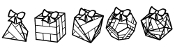Christmas card 2017Christmas card 2016

Comments in green were written by me. Comments in blue were not written by me.
@JDev: lots of the card will still be brown once you're done, but you should see a nice picture. Perhaps one of your answers is wrong, making a mess of the picture?
Matthew
I finished all of the puzzles but the picture is far from colored in. Am I missing something?

These puzzles have been a blast!
JDev
@Tara: I initially made the same mistake. Maybe you didn't take into account that 6 is not one of the available digits in question 1?
Sean
@Tara: Yes, looks like you may have got an incorrect answer for one of the black puzzles
Matthew

Allowed HTML tags: <br> <a> <small> <b> <i> <s> <sup> <sub> <u> <spoiler> <ul> <ol> <li>
To prove you are not a spam bot, please type "sexa" backwards in the box below (case sensitive):
2020-11-22
This year, the front page of mscroggs.co.uk will once again feature an Advent calendar, just like in the five previous Decembers. Behind each door, there will be a puzzle with a three digit solution. The solution to each day's puzzle forms part of a logic puzzle:
It's nearly Christmas and something terrible has happened: you've just landed in a town in the Arctic circle with a massive bag of letters for Santa, but you've lost to instructions for how to get to Santa's house near the north pole. You need to work out where he lives and deliver the letters to him before Christmas is ruined for everyone.
Due to magnetic compasses being hard to use near the north pole, you brought with you a special Advent compass. This compass has nine numbered directions. Santa has given the residents of the town clues about a sequence of directions that will lead to his house; but in order to keep his location secret from present thieves, he gave each resident two clues: one clue is true, and one clue is false.
The residents' clues will reveal to you a seqeunce of compass directions to follow. You can try out your sequences on this map.
Behind each day (except Christmas Day), there is a puzzle with a three-digit answer. Each of these answers forms part of a resident's clue. You must use these clues to work out how to find Santa's house.
Ten randomly selected people who solve all the puzzles, find Santa's house, and fill in the entry form behind the door on the 25th will win prizes!
The winners will be randomly chosen from all those who submit the entry form before the end of 2020. Each day's puzzle (and the entry form on Christmas Day) will be available from 5:00am GMT. But as the winners will be selected randomly, there's no need to get up at 5am on Christmas Day to enter!
To win a prize, you must submit your entry before the end of 2020. Only one entry will be accepted per person. If you have any questions, ask them in the comments below or on Twitter.
So once December is here, get solving! Good luck and have a very merry Christmas!

### Similar postsChristmas (2020) is overChristmas (2019) is overChristmas (2019) is coming!Christmas (2018) is over

Comments in green were written by me. Comments in blue were not written by me.
Nice one today (16 December) :)
Did he?... did he really?... starts to look like it... yes he did! :D
Gert-Jan
@A: Aha, I should have thought of this! Puzzles completed :-)
(anonymous)
@Dean: you can also go through each answer one at a time and change a digit; if the number of wrong answers goes up then your answer for that question was correct
A
@Dean: Yes, I'm planning to change how that bit works. Check back tomorrow or the next day for a more fun finish!
Matthew
A bit harsh that we can’t tell exactly which answers are wrong! I won’t have time to revisit every puzzle - and its kind of less fun redoing something that is already correct... :-(
Dean
@Marty: Yes, I'm in the middle of correcting the clues page to add these details back
Matthew

Allowed HTML tags: <br> <a> <small> <b> <i> <s> <sup> <sub> <u> <spoiler> <ul> <ol> <li>
To prove you are not a spam bot, please type "d" then "e" then "c" then "a" then "g" then "o" then "n" in the box below (case sensitive):
2020-07-29
A week ago, it was 22 July: Pi Approximation Day. 22/7 (22 July in DD/M format) is very close to pi, closer in fact than 14 March's approximation of 3.14 (M.DD).
During this year's Pi Approximation Day, I was wondering if there are other days that give good approximations of interesting numbers. In particular, I wondered if there is a good 2π (or τ) approximation day.
π is close to 22/7, so 2π is close to 44/7—but sadly there is no 44th July. The best approximation day for 2π is 25th April, but 25/4 (6.25) isn't really close to 2π (6.283185...) at all. The day after Pi Approximation Day, however, is a good approximation of 2π-3 (as π-3 is approximately 1/7). After noticing this, I realised that the next day would be a good approximation of 3π-6, giving a nice run of days in July that closely approximate expressions involving pi.
After I tweeted about these three, Peter Rowlett suggested that I could get a Twitter bot to do the work for me. So I made one: @HappyApproxDay.
@HappyApproxDay is currently looking for days that approximate expressions involving π, τ, e, √2 and √3, and approximate the chosen expression better than any other day of the year. There are an awful lot of ways to combine these numbers, so @HappyApproxDay looks like it might be tweeting quite a lot...

### Similar postsLogic bot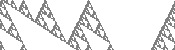Close encounters of the second kindChristmas (2020) is overChristmas card 2020

Comments in green were written by me. Comments in blue were not written by me.